Type
Solution Manual
Book Title
Fundamentals of Investments: Valuation and Management 8th Edition
ISBN 13
978-1259720697

### 978-1259720697 Chapter 10 Solution Manual

January 2, 2020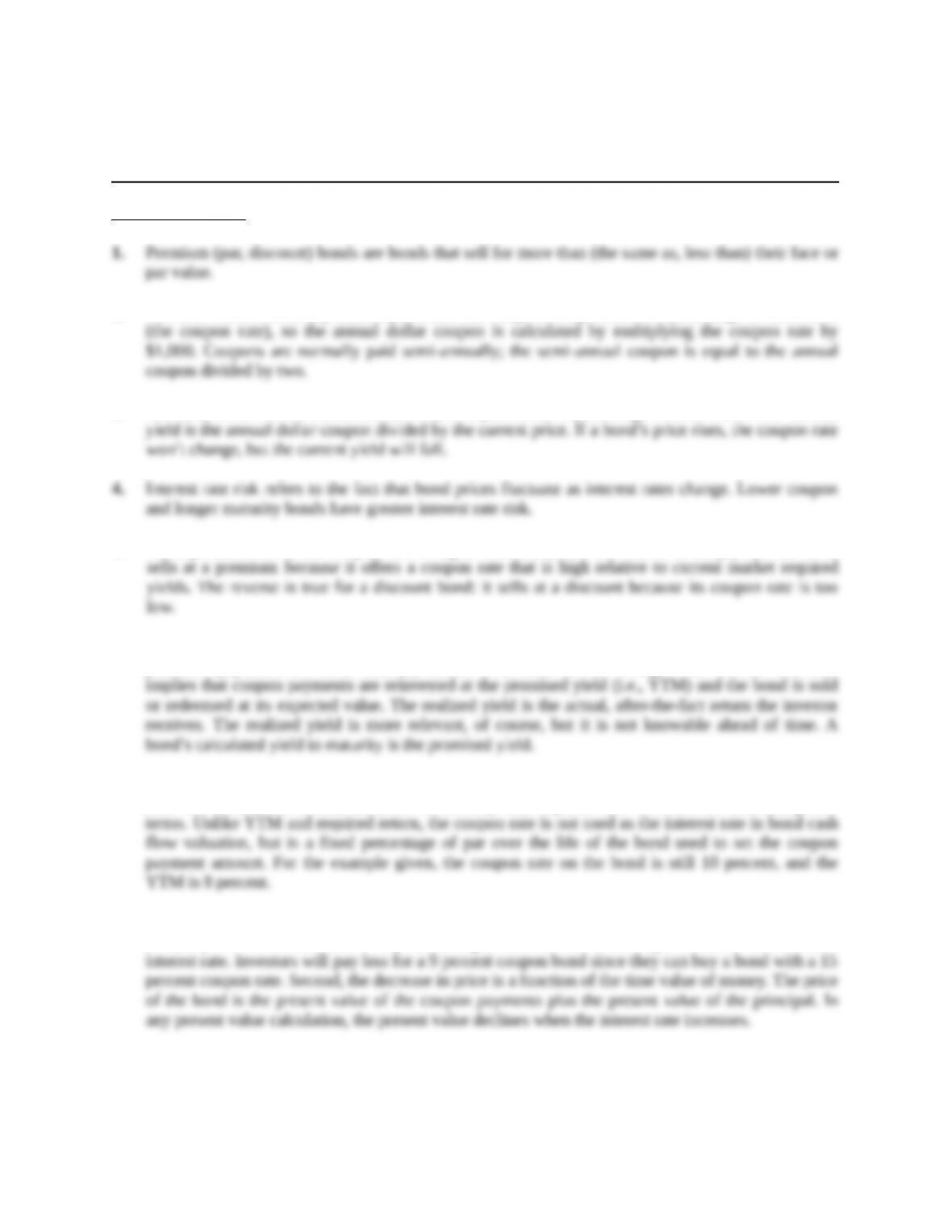Chapter 10
Bond Prices and Yields
Concept Questions
2. The face value is normally \$1,000 per bond. The coupon is expressed as a percentage of face value
3. The coupon rate is the annual dollar coupon expressed as a percentage of face value. The current
5. For a premium bond, the coupon rate is higher than the yield. The reason is simply that the bond
6. A bond’s promised yield is an indicator of what an investor can expect to earn if (1) all of the bond’s
promised payments are made and (2) market conditions do not change. This second condition
7. The yield to maturity is the required rate of return on a bond expressed as a nominal annual interest
rate. For noncallable bonds, the yield to maturity and required rate of return are interchangeable
8. Since the yield increased, the price of the bond will decrease. This can be explained in two ways.
First, any new bonds will have a 15 percent coupon rate in order to sell at par since that is the market
Education.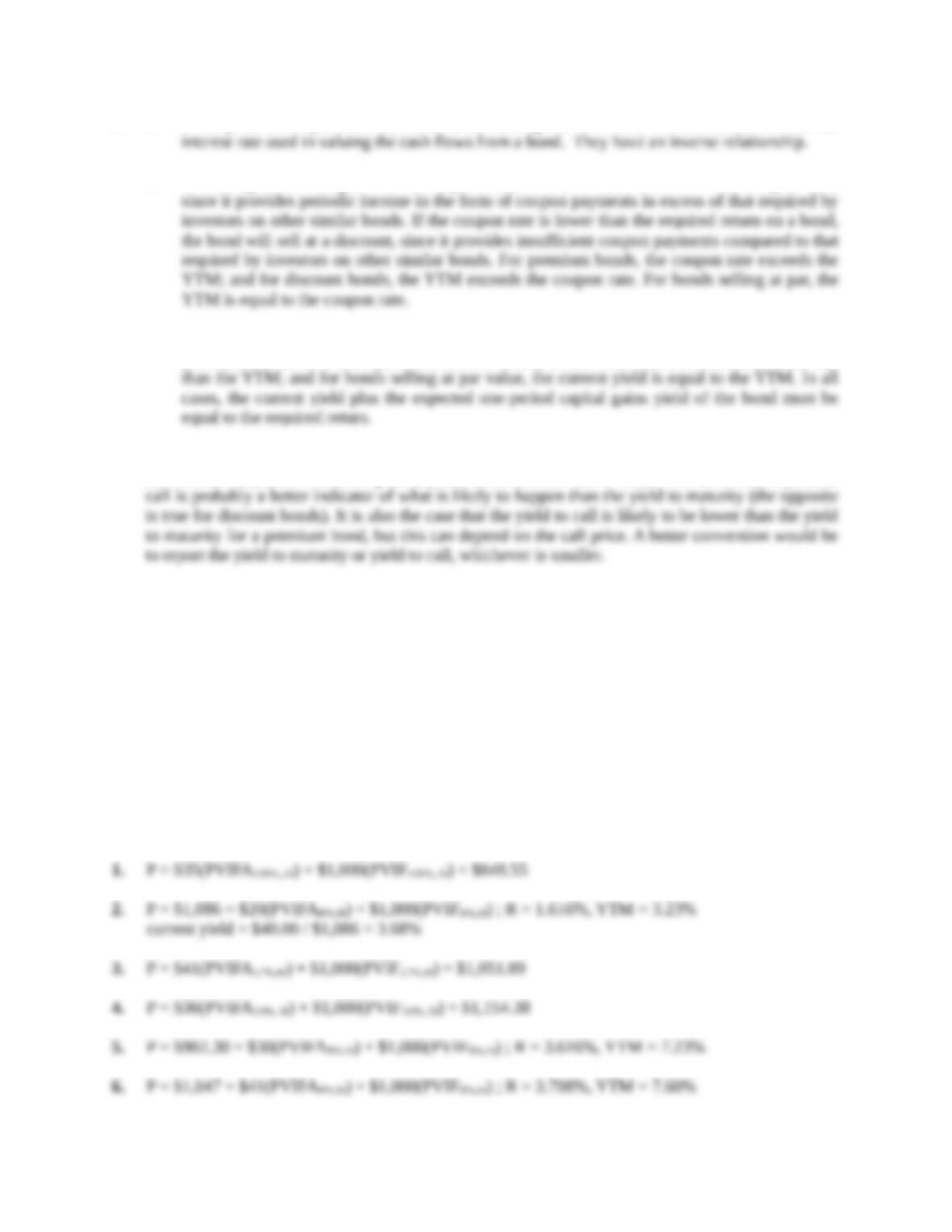9. a. Bond price is the present value term when valuing the cash flows from a bond; YTM is the
b. If the coupon rate is higher than the required return on a bond, the bond will sell at a premium,
c. Current yield is defined as the annual coupon payment divided by the current bond price. For
premium bonds, the current yield exceeds the YTM; for discount bonds the current yield is less
10. A premium bond is one with a relatively high coupon, and, in particular, a coupon that is higher than
current market yields. These are precisely the bonds that the issuer would like to call, so a yield to
Solutions to Questions and Problems
NOTE: All end of chapter problems were solved using a spreadsheet. Many problems require multiple
steps. Due to space and readability constraints, when these intermediate steps are included in this
solutions manual, rounding may appear to have occurred. However, the final answer for each problem is
found without rounding during any step in the problem.
Also note that we have written out solutions using formulas; however, students should consult section
10.3 in the book for a detailed example on how to compute price and yield using a financial calculator.
This can easily be done for each problem as we have identified the relevant input variables in each
formula.
Core Questions
Education.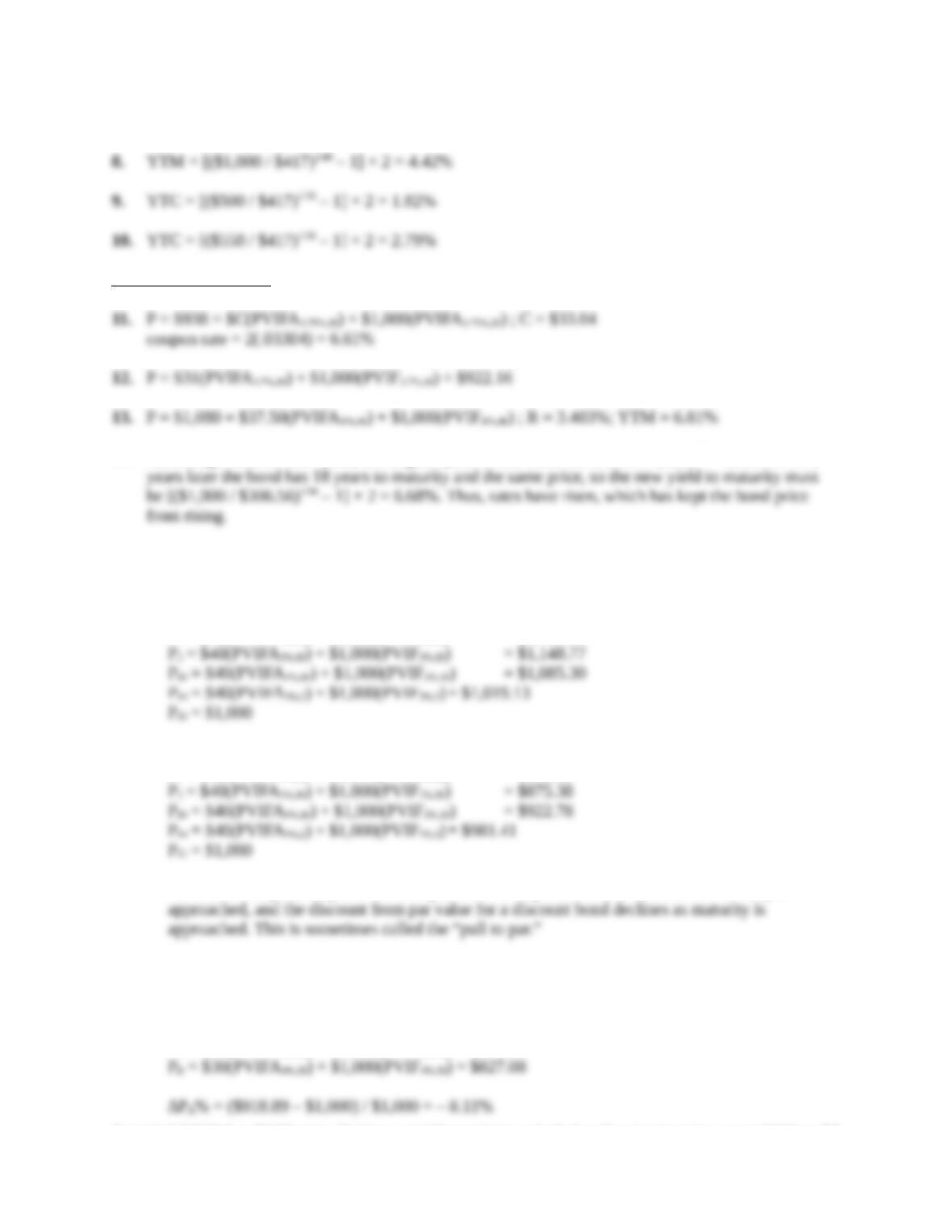7. P = \$928 = \$37.50(PVIFAR%,18) + \$1,000(PVIFR%,18) ; R = 4.334%; YTM = 8.67%
Intermediate Questions
14. Assuming a \$1,000 face value, the original price of the bond was \$1,000 / (1.03)40 = \$306.56. Two
15. If held to maturity, a zero-coupon bond will always have a realized yield equal to its original yield to
maturity, which in this case is 6 percent.
16. P: P0 = \$40(PVIFA3%,30) + \$1,000(PVIF3%,30) = \$1,196.00
P1 = \$40(PVIFA3%,28) + \$1,000(PVIF3%,28) = \$1,187.64
D: P0 = \$40(PVIFA5%,30) + \$1,000(PVIF5%,30) = \$846.28
P1 = \$40(PVIFA5%,28) + \$1,000(PVIF5%,28) = \$851.02
All else held equal, the premium over par value for a premium bond declines as maturity is
17. If both bonds sell at par, the initial YTM on both bonds is the coupon rate, 6 percent. If the YTM
suddenly rises to 8 percent, then:
PA = \$30(PVIFA4%,10) + \$1,000(PVIF4%,10) = \$918.89
Education.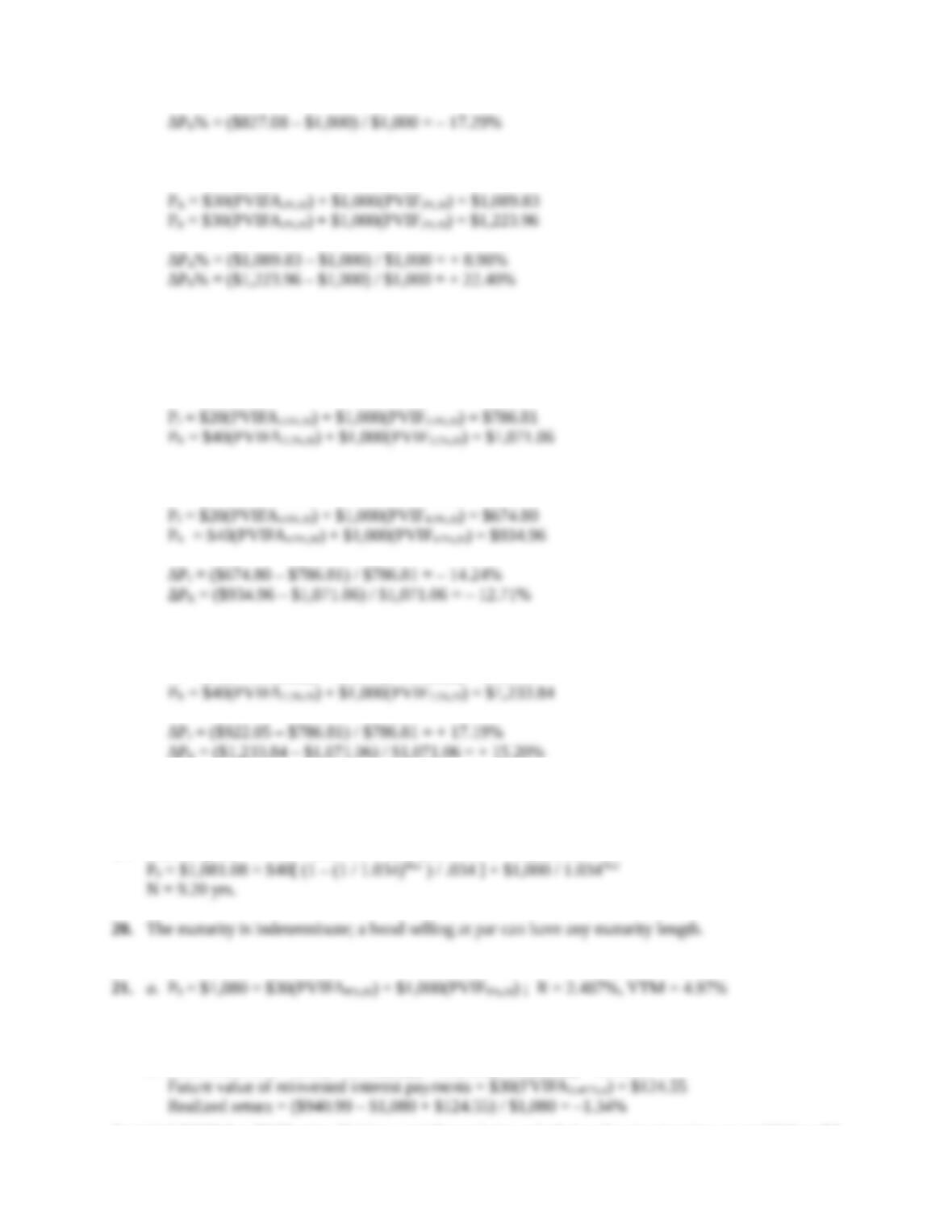If the YTM suddenly falls to 4 percent, then:
All else the same, the longer the maturity of a bond, the greater is its price sensitivity to changes
in interest rates.
18. Initially, at a YTM of 7 percent, the prices of the two bonds are:
If the YTM rises from 7 percent to 9 percent:
If the YTM declines from 7 percent to 5 percent:
PJ = \$20(PVIFA2.5%,20) + \$1,000(PVIF2.5%,20) = \$922.05
∆PK = (\$1,233.84 – \$1,071.06) / \$1,071.06 = + 15.20%
All else the same, the lower the coupon rate on a bond, the greater is its price sensitivity to
changes in interest rates.
19. Current yield = .0740 = \$80 / P0 ; P0 = \$80 / .0740 = \$1,081.08
This is the rate of return you expect to earn on your investment when you purchase the bond.
b. Price when sold = \$30(PVIFA3.487%,16) + \$1,000(PVIF3.487%,16) = \$940.99
Education.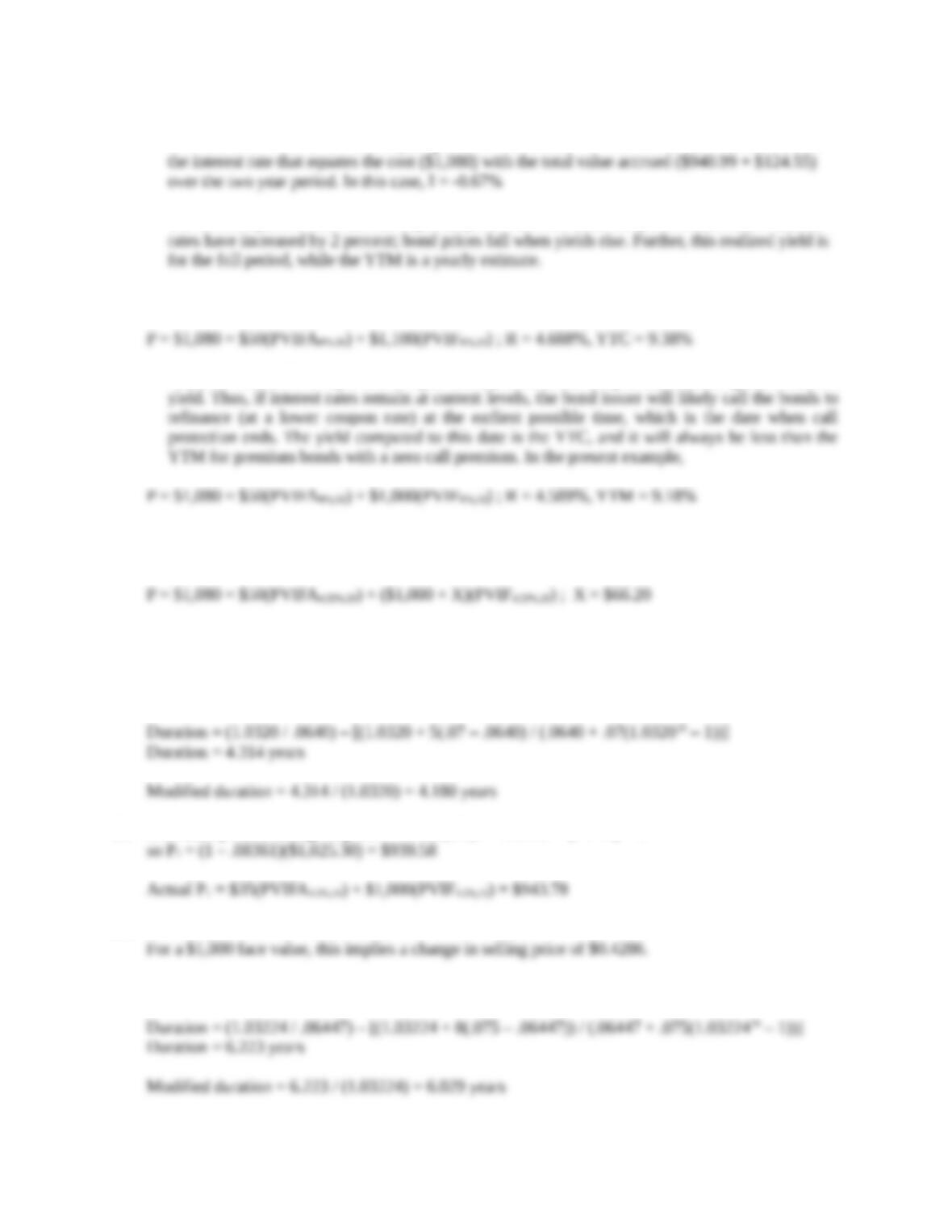This is a total return over the two year period. If we want an annual number we would calculate
The realized yield is less than the expected yield when the bond was purchased because interest
22. The yield to call can be computed as:
Since the bond sells at a premium to par value, you know the coupon rate must be greater than the
where if the bond is held until maturity, no call premium must be paid. Note that using the same
analysis, a break-even call premium can also be computed:
Thus, if interest rates remain unchanged, the bond will not be called if the call premium is greater
than \$66.20.
23. P = \$1,025.30 = \$35(PVIFAR%,10) + \$1,000(PVIFR%,10) ; R = 3.20%, YTM = 6.40%
24. Estimated percent change in price = –4.180(.02) = –.08361 = (P1 / P0) – 1
25. Dollar value of an 01 = 4.18 × \$102.53 × .0001 = .0429
26. P = \$1,065 = \$37.50(PVIFAR%,16) + \$1,000(PVIFR%,16) ; R = 3.224%, YTM = 6.447%
Education.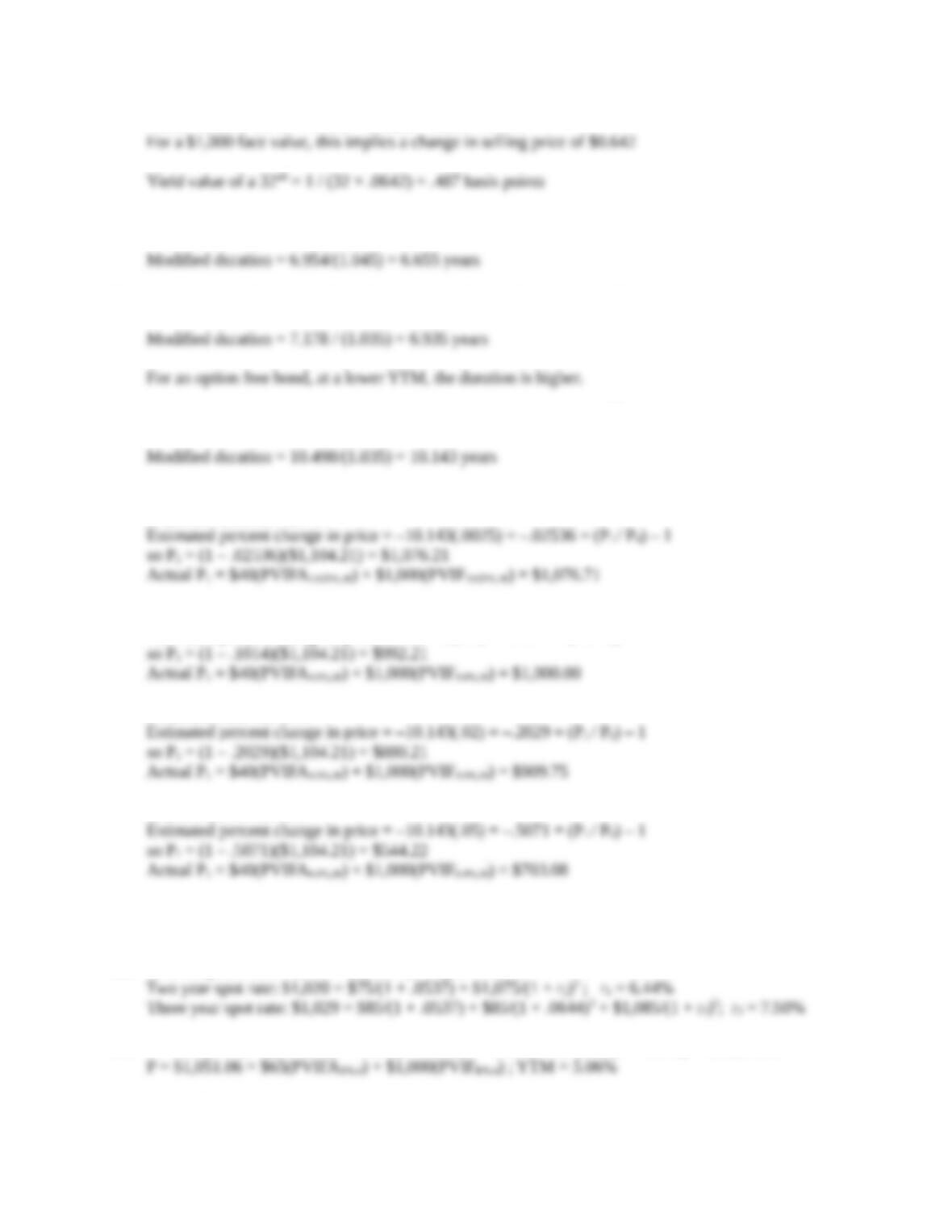Dollar value of an 01 = 6.029 × \$106.5 × .0001 = .0642
27. Duration = (1.045 / .09) – [(1.045 + 10(.08 – .09)) / (.09 + .08(1.04520 – 1))] = 6.954 years
28. Duration = (1.035 / .07) – [(1.035 + 10(.08 – .07)) / (.07 + .08(1.03520 – 1))] = 7.178 years
29. Duration = (1.035 / .07) – [(1.035 + 19(.08 – .07)) / (.07 + .08(1.03538 – 1))] = 10.498 years
30. Initial price = \$40(PVIFA3.5%, 38) + \$1,000(PVIF3.5%,38) = \$1,104.21
If interest rates rise .25%:
If interest rates rise 1%:
Estimated percent change in price = –10.143(.01) = –.1014 = (P1 / P0) – 1
If interest rates rise 2%:
If interest rates rise 5%:
While duration gives an effective estimate for small interest rate changes, duration does not produce
a good estimate of the price change for large interest rate changes.
31. Zero coupon YTM = \$949 = \$1,000 / (1 + r); r = 5.37%
32. P = \$65/(1 + .0420) + \$65/(1 + .0450)2 + \$65/(1 + .0490)3 + \$1,065/(1 + .0510)4 = \$1,051.06
Education.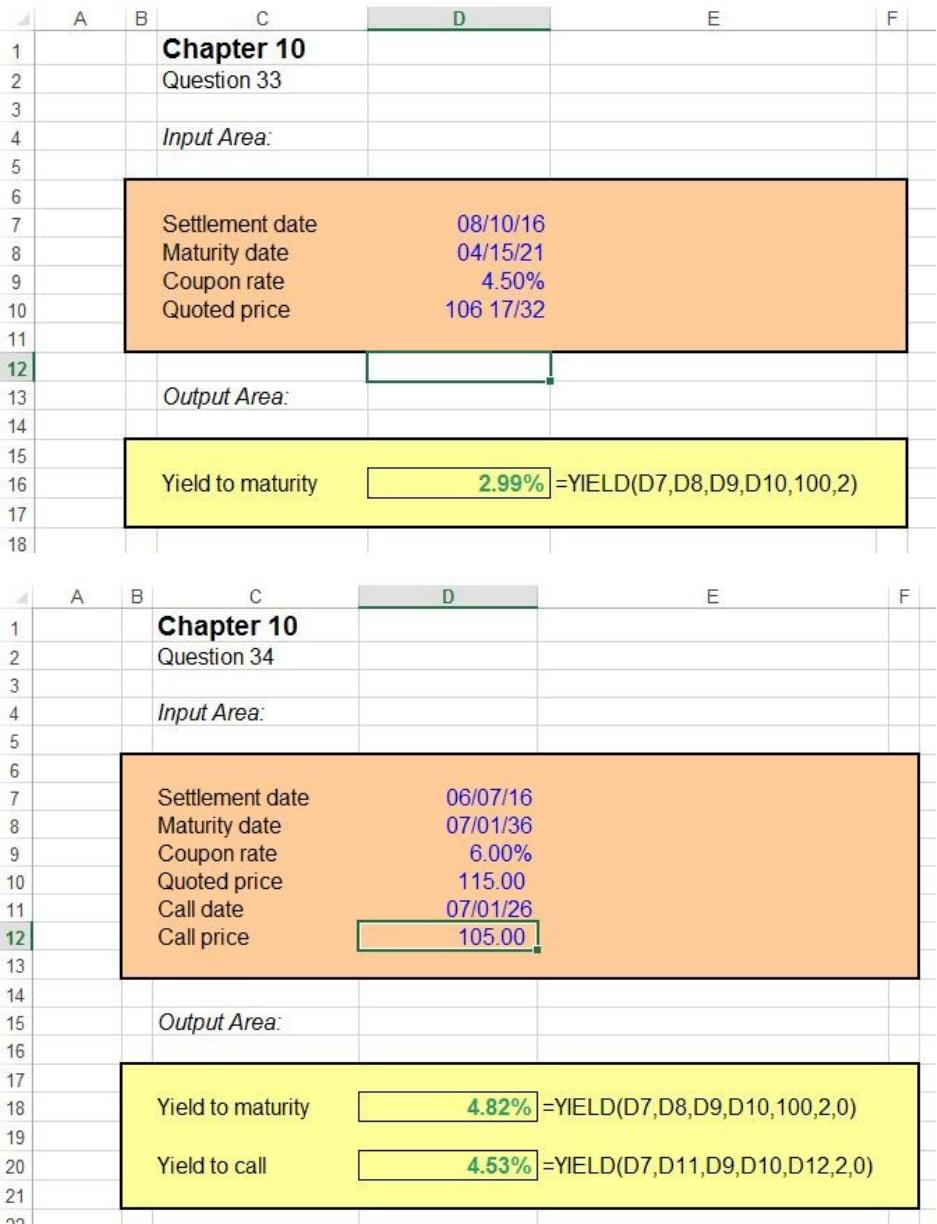Education.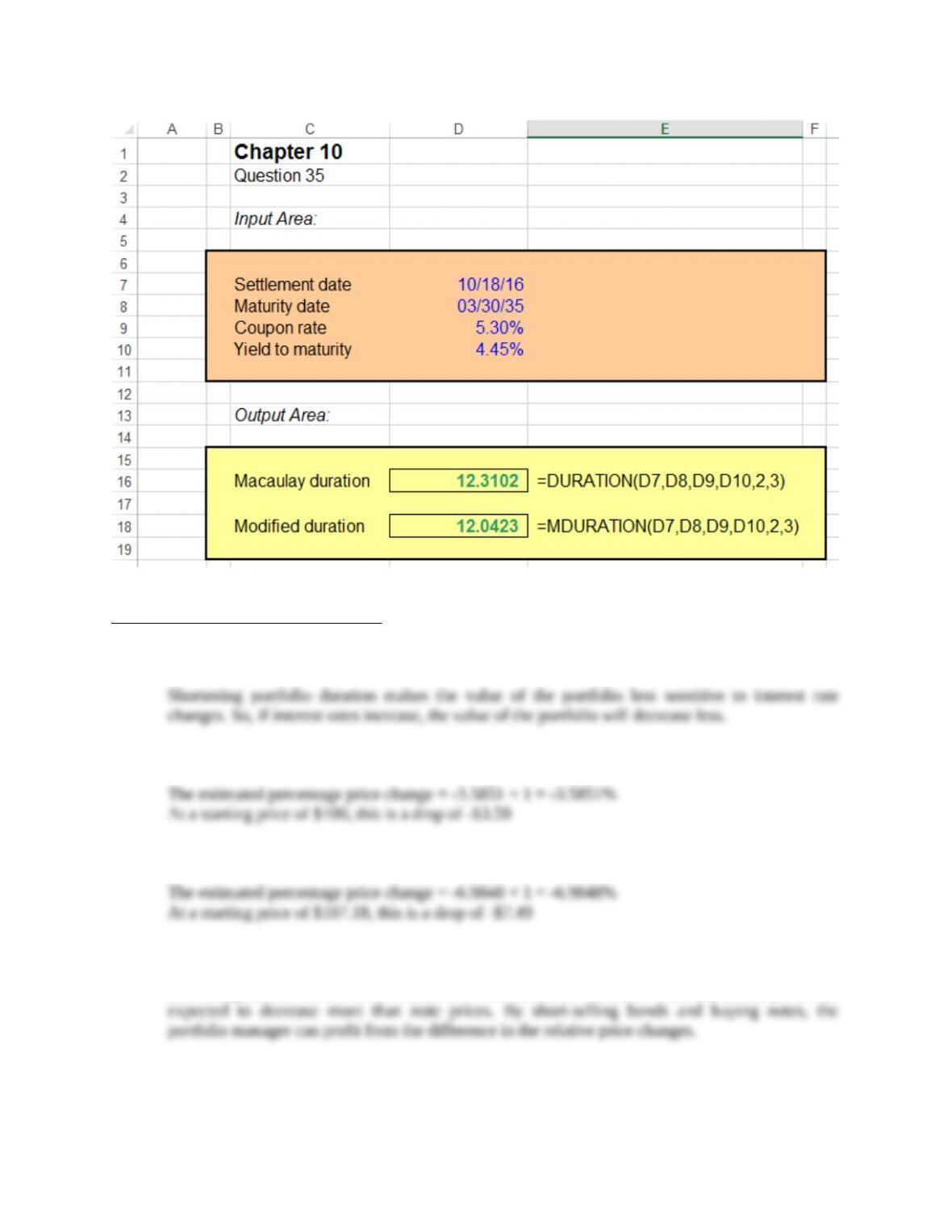CFA Exam Review by Kaplan Schweser
1. c
2. b
3. a
4. a
Since long-term interest rates are expected to increase more than short-term rates, bond prices are